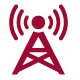# Applied Techniques for Economists

 Module code: ECON 1541 Module description This module is designed to teach students the key mathematical and statistical techniques that will enable them to understand the models and arguments used within micro and macroeconomic theory and application. The course will divided into two sections, starting with an introduction to the basic mathematical concepts such as elasticity used in demand and supply models and maximising profit functions: The Straight Line, Mathematical Modelling and Applications in Economics Translations of Linear Functions, Elasticity, Budget and Cost Constraints Solving Simultaneous Linear Equations (determining points of equilibrium) Analysis of cost and revenue functions Non Linear Relationship: Production functions, growth models Calculus for optimization and value measurement (consumer surplus) The second part of the course will comprise of an introduction to statistics methods and their use in making sense of the economic data that students will use in an applied context: Introduction to Statistics: Applications in Finance and Economics, Data and Data Sources Summarising Data, Exploratory Data Analysis, and Graphical Representation Measures of Location, (mean median mode) Variability (standard deviation, detection of outliers and measures of association between variables Sampling theory and estimation and hypothesis testing Correlation and simple regression Contact hours per student per year Workshops: 40 hours (lectures and classes are combined into workshops) Assessments Assignment (1000 words): 50% Exam (60 mins): 50% Additional Costs: No extra costs other than purchase of books and a scientific calculatorAt DMU there is always something to do or see, check out our events for yourself.DMU is a dynamic university, read about what we have been up to in our latest news section.Read about our mission and vision and how these create a supportive and exciting learning environment.